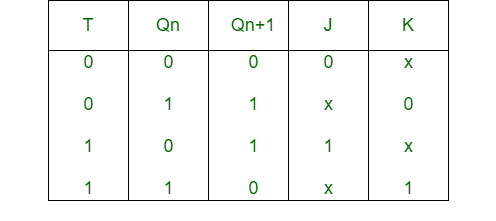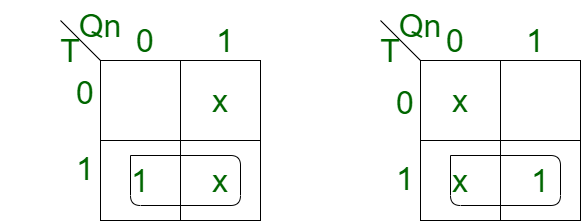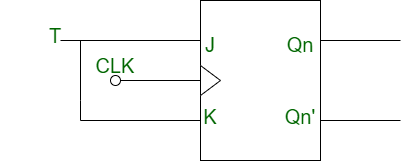# Conversion of J-K Flip-Flop into T Flip-Flop

Prerequisite – Flip-flop

1. J-K Flip-Flop: JK flip-flop shares the initials of Jack Kilby, who won a Nobel prize for his fabrication of the world’s first integrated circuit, some people speculate that this type of flip flop was named after him because a  flip-flop was the first device that Kilby build when he was developing integrated circuits. J-K flip-flop is the gated version of SR flip-flop with an addition of extra input i.e. clock input. It prevents invalid output conditions when both the inputs are at the same value.

2. T Flip-Flop: T flip-flop means Toggle flip-flop. It changes the output on each clock edge and gives an output that is half the frequency of the signal to the input.

### Conversion of J-K Flip-Flop into T Flip-Flop:

• Step-1: Construct the characteristic table of T flip-flop and excitation table of the J-K flip-flop.• Step-2: Using the K map, find the boolean expression for J and K in terms of T.```J = T
K = T ```
• Step-3: Construct the circuit diagram for the conversion of the J-K flip-flop into a T flip-flop.